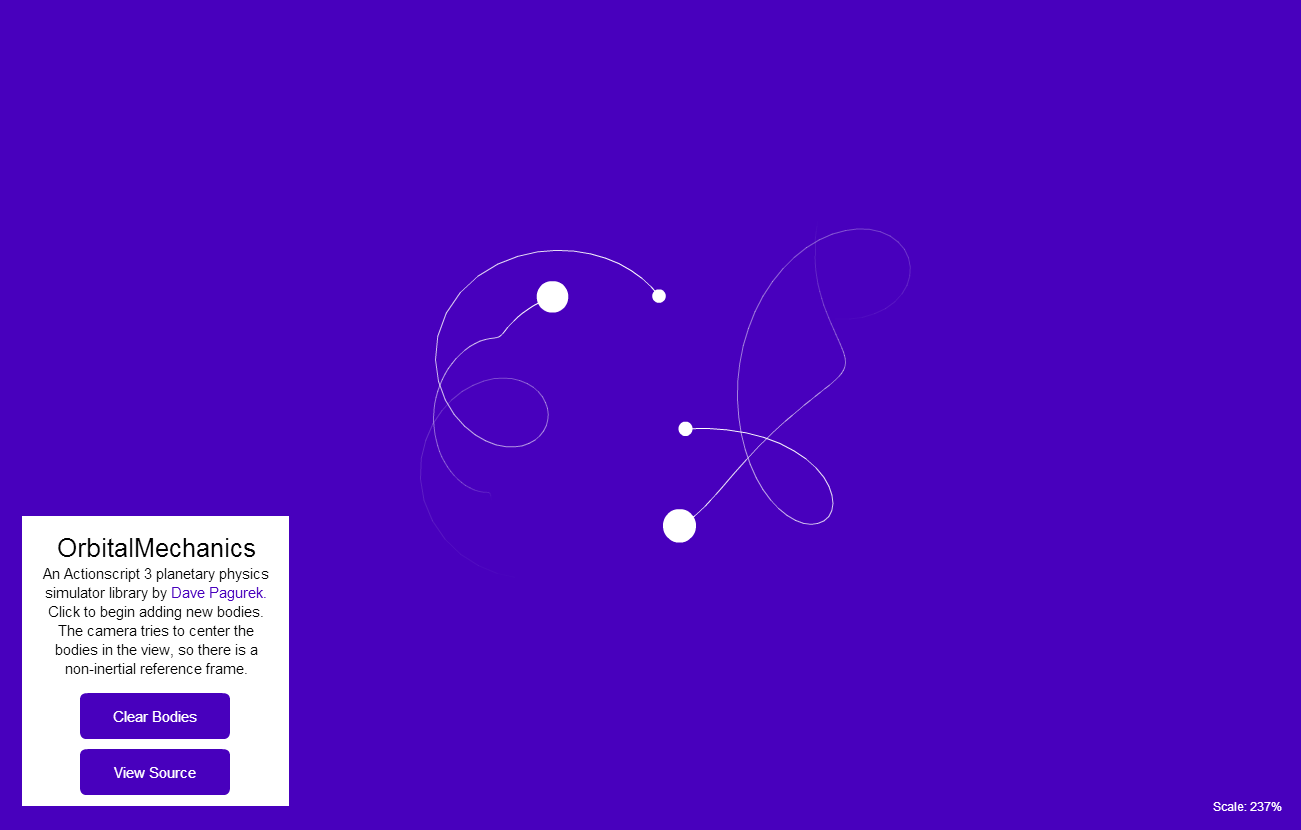# OrbitalMechanics

January 4, 2014

OrbitalMechanics is a planetary physics simulator written in Actionscript 3.

Basically, it is an application of various parts of the Grade 12 physics curriculum. Gravitational force is calculated using the formula F = (G m1 m2)/(d2), where G is the arbitrary number 100, masses are in arbitrrary units where mass = 1 is comparable to a small asteroid, and distance is measured in Flash units. When two bodies collide (when the distance between them is less than the sum of their radii), they are replaced with a single body with their combined mass. The resulting body's velocity is found assuming it is a perfectly inelastic collision using the formula m1v1 + m2v2 = (m1 + m2)v'.

I chose to program this in Actionscript 3 because although Flash as a web medium is on the decline, I still believe that Actionscript 3 is a real pleasure to work with. Actionscript has a vector graphics library, which in itself is an improvement over HTML5 canvas. All the language-related issues I had with AS2 were, in my opinion, fixed in AS3. It's object-oriented and event-based, and larger projects are much more manageable. It's great, and since I was really only making OrbitalMechanics for fun, I decided not to worry about using more future-proof technology.

## Features

• Calculates gravitational force
• Simulates inelastic collisions
• Provides example UI

## Example

Here is an example body with an initial velocity being added to the system:

``````var system:OrbitalMechanics = new OrbitalMechanics();
system.addBody(new Body(0, 50, 3, new Geovector(6, Math.PI)));
system.x = 0;
system.y = 0;